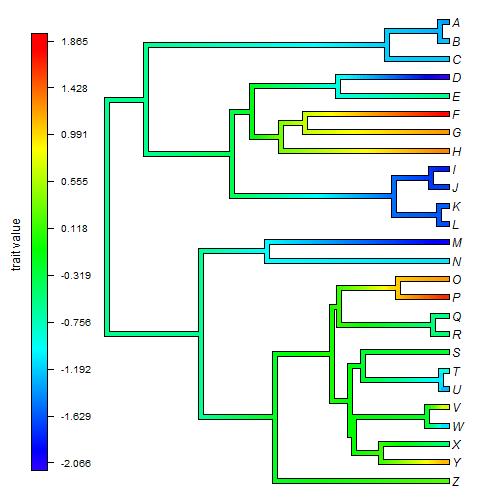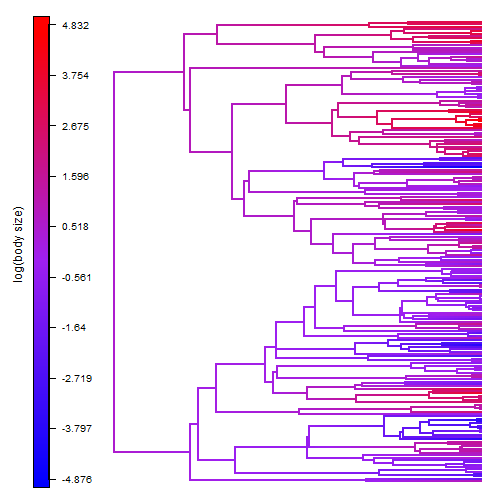## Wednesday, August 17, 2016

### Vertical legend in `"contMap"` style plots

I recently updated the mostly internally used phytools function, `add.color.bar`, that is employed by plot methods `plot.contMap` & `plot.densityMap`, and can be used with `plotBranchbyTrait` (for instance), to permit the color gradient bar to be plotted vertically in either an upwards or downwards direction (1, 2).

The default behavior of this function is not particularly interesting, but below I show how it can be used to create a vertical color legend for a plotted `"contMap"` option:

``````library(phytools)
## create "contMap" object without plotting it
obj<-contMap(tree,x,plot=FALSE)
## invert color map
obj<-setMap(obj,invert=TRUE)
## now let's plot our "contMap" object & create a legend
h<-max(nodeHeights(obj\$tree))
plot(obj,legend=FALSE,xlim=c(-0.25*h,1.1*h))
## now add our legend, but without the text underneath
lims=NULL,digits=3,direction="upwards",
subtitle="",lwd=15,x=-0.2*h,y=2,prompt=FALSE)
## get line width in user units
LWD<-diff(par()\$usr[1:2])/dev.size("px")
lines(x=rep(-0.2*h+LWD*15/2,2),y=c(2,Ntip(obj\$tree)-1))
nticks<-10
Y<-cbind(seq(2,Ntip(obj\$tree)-1,length.out=nticks),
seq(2,Ntip(obj\$tree)-1,length.out=nticks))
X<-cbind(rep(-0.2*h+LWD*15/2,nticks),
rep(-0.2*h+LWD*15/2+0.02*h,nticks))
for(i in 1:nrow(Y)) lines(X[i,],Y[i,])
ticks<-seq(obj\$lims,obj\$lims,length.out=nticks)
text(x=X[,2],y=Y[,2],round(ticks,3),pos=4,cex=0.8)
``````Pretty neat.

With some tweaks - for instance, changing the font size and line widths - this can also be applied to much larger trees:

``````obj<-contMap(big.tree,y,plot=FALSE)
obj<-setMap(obj,colors=c("blue","purple","red"))
h<-max(nodeHeights(obj\$tree))
plot(obj,legend=FALSE,xlim=c(-0.25*h,h),lwd=2,
outline=FALSE,ftype="off")
lims=NULL,digits=3,direction="upwards",
subtitle="",lwd=15,x=-0.2*h,y=2,prompt=FALSE)
## get line width in user units
LWD<-diff(par()\$usr[1:2])/dev.size("px")
lines(x=rep(-0.2*h+LWD*15/2,2),y=c(2,Ntip(obj\$tree)-1))
nticks<-10
Y<-cbind(seq(2,Ntip(obj\$tree)-1,length.out=nticks),
seq(2,Ntip(obj\$tree)-1,length.out=nticks))
X<-cbind(rep(-0.2*h+LWD*15/2,nticks),
rep(-0.2*h+LWD*15/2+0.02*h,nticks))
for(i in 1:nrow(Y)) lines(X[i,],Y[i,])
ticks<-seq(obj\$lims,obj\$lims,length.out=nticks)
text(x=X[,2],y=Y[,2],round(ticks,3),pos=4,cex=0.8)
``````One of the more challenging issues that I ran into was (surprisingly) figuring out how to get the line width in user units! I need this value in order to correctly place my tick marks on the color gradient legend. In the end, I figured out I could just get the device width in pixels using `dev.size("px")`, and then devide this quantity by the device width in user units, `diff(par()\$usr[1:2])`. Since a line width of `lwd=1` is one pixel wide, this quantity multiple by the `lwd` for the gradient bar should be the width of that bar in user units.

This update can be obtained by pulling down the latest version of phytools from GitHub:

``````library(devtools)
install_github("liamrevell/phytools")
``````

The data for this exercise were simulated using phytools as follows:

``````## example 1
tree<-pbtree(n=26,tip.label=LETTERS[26:1])
x<-fastBM(tree)
## example 2
big.tree<-pbtree(n=200)
y<-fastBM(big.tree)
``````

1.Wonderful, thanks Liam!!!

2.So cool, thanks Liam!

I was using contMap just right now for a paper and wanted to ask you something about it (and I will use this update).

I would like to use contMap as a tool for visualization of results, but I have a very small phylogeny (5 taxa). Do you think is justified to use contMap with the only purpose of visualization? Or it will be critizised anyway because of the sample size?

Thanks for all the work in this blog, is amazing always.
Cheers,
Borja

Note: due to the very large amount of spam, all comments are now automatically submitted for moderation.